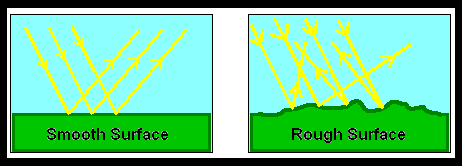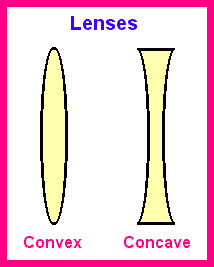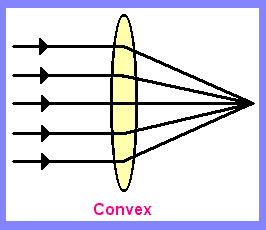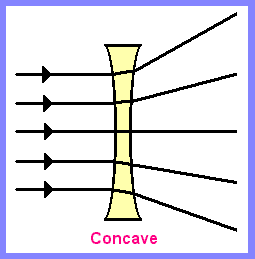# Reflection & Refraction

Reflection & Refraction Light Lesson - Elementary Science Lessons & Worksheets - My Schoolhouse

 Reflection occurs when light bounces off objects.  How much reflection depends upon how even the surface is.  If the surface is rough, the light scatters.  If the surface is smooth and flat, the light will bounce off it at equal angles.  That is why a flat mirror reflects a good likeness of the object being reflected. Look at the diagrams below.  Notice the angles at which the rays of light strike the surfaces.Refraction occurs because light bends.  A lens is a piece of transparent material.  It is usually made of glass and has at least one curved surface. Look at the convex and concave lenses below.The curved surface/surfaces of a lens bends the light.  Notice the paths of light of the convex and concave lenses shown below.Directions: Answer the questions about reflection and refraction. occurs when light bounces off an object. occurs when light is bent. When light strikes a surface that is rough, the light will  . When light strikes a surface that is smooth, the light will bounce off at equal . What type of material is a lens? transparent translucent opaque   The path of light through a concave lens is not directed to a single focal point directed to a single focal point   The path of light through a convex lens is not directed to a single focal point directed to a single focal point

 Thank You for using My Schoolhouse